## 3641 Reputation

7 years, 362 days

## why Maple did this automatic simplificat...

Maple 2020

One thing I always liked about Maple, is that it does not do automatic simplifications or automatic re-write of expressions without the user explicitly asking for it like some other CAS systems do, which I think this is the right way.

So I was surprised when I set up a rational expression of a numerator and denominator, then when I asked for its denom and numer, I found Maple did automatic rewrite (even though on the screen the expression remained as it was originally). This caused a bug in my program (since it assumed as long as no simplifcation is made on the expression, numer and denom will remain as it was orginally placed). Here is an example

```restart;
num:=-(1-x/exp(y));
den:=(exp(y)+x);
expr:=num/den;
```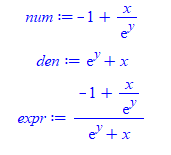So now, one would expect, by looking at the screen, when asking for numer(expr) to obtain back -(1-x/exp(y))  but this is not what happens

`numer(expr)`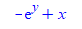And

`denom(expr)`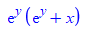So Maple's internal representation of numer and denom, is not what "appears" on the screen for the user. Maple automatically replaced -1+x/exp(y) by (-exp(y)+x)/exp(y) and then moved denominator of this, which is exp(y), down to the denominator of the orginal expression.

I was surprised by this, since as I said, my experence with Maple is that it keeps expressions as entered and will change them only when the user asks for a change.

How common is such behavior in Maple? and why did Maple do this under the cover manipulation in this case? It is probably documented somewhere if I search hard enough.

## dsolve gives solution with no y(x) in i...

Maple 2020

I was trying to compare my solution with Maple on this ode, when I noticed Maple gives solution with no y(x)= in it.

```restart;
ode:=(y(x)-x*diff(y(x),x))/(y(x)^2+diff(y(x),x))=(y(x)-x*diff(y(x),x))/(1+x^2*diff(y(x),x));
sol:=dsolve(ode)
```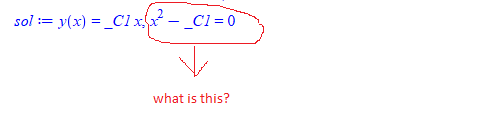When there is more than one solution, Maple write y(x) on each. For an example

`dsolve(diff(y(x),x)^2=x)`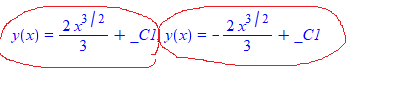How to explain this output?

Maple 2020.1

## another problem with odetest. Verificati...

Maple 2020

I think I've seen such a problem before but thought it was fixed.

Here is an example, where depending on what one calls the constant of integration, maple can or not, verify the solution of the ode.

This should not happen.

When calling the constant of integration C or _C Maple does not verify the solution. Only when using _C1 it does.

This is a problem, since I do not use _C1 or _C2  in my solution (so not to conflict with Maple's), I use C and C.

Is there a workaround for this?

Example 1

```ode:=diff(z(x),x)+z(x)*cos(x)=z(x)^n*sin(2*x);
mysol:=z(x) = 1/((_C1*exp(sin(x)*(n - 1))*n - _C1*exp(sin(x)*(n - 1)) + 2*sin(x)*n - 2*sin(x) + 2)/(n - 1))^(1/(n - 1));
odetest(mysol,ode);

0```

Now I just changed _C1 to C

```restart;
ode:=diff(z(x),x)+z(x)*cos(x)=z(x)^n*sin(2*x);
mysol:=z(x) = 1/(( C*exp(sin(x)*(n - 1))*n - C*exp(sin(x)*(n - 1)) + 2*sin(x)*n - 2*sin(x) + 2)/(n - 1))^(1/(n - 1));
odetest(mysol,ode);
```

and I get 2 pages full of stuff thrown at the screen. Same when using C as constant of integration.

I could change my C and C to _C1 and _C2 each time just before calling odetest as workaround.

But would like to ask if this is supposed to happen and why it happens. I depend on odetest alot, as I use it to tell me if my solution is correct or not and I do not want false negatives.

I have found additional ones. May be these will help locate the problem

Example 2

```restart;
ode:=diff(y(x),x) = (1+x+y(x))^(1/2);
mysol1:=y(x) = LambertW(-exp(-x/2 - 1 + C/2))^2 + 2*LambertW(-exp(-x/2 - 1 + C/2)) - x:
odetest(mysol1,ode);
```

gives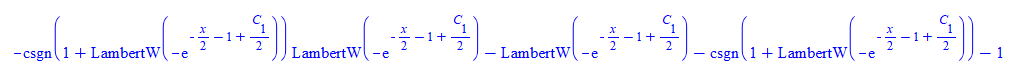```restart;
ode:=diff(y(x),x) = (1+x+y(x))^(1/2):
mysol2:=y(x) = LambertW(-exp(-x/2 - 1 + _C1/2))^2 + 2*LambertW(-exp(-x/2 - 1 + _C1/2)) - x:
odetest(mysol2,ode);
```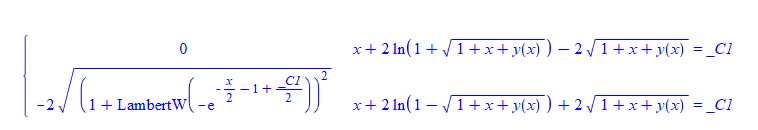Example 3

```restart;
ode:=diff(y(x),x) = f(x)*y(x)+g(x)*y(x)^k:
mysol1:=y(x) = (-exp(-(k - 1)*Int(f(x), x))*(int(g(x)*exp((k - 1)*Int(f(x), x)), x)*k - int(g(x)*exp((k - 1)*Int(f(x), x)), x) - C))^(-1/(k - 1)):
odetest(mysol1,ode);

#long output is given```

Changing C to _C1 gives 0 right away

```restart;
ode:=diff(y(x),x) = f(x)*y(x)+g(x)*y(x)^k:
mysol2:=y(x) = (-exp(-(k - 1)*Int(f(x), x))*(int(g(x)*exp((k - 1)*Int(f(x), x)), x)*k - int(g(x)*exp((k - 1)*Int(f(x), x)), x) - _C1))^(-1/(k - 1)):
odetest(mysol2,ode);

0```

Example 4

```restart;
ode:=3*y(x)*diff(y(x),x)+5*cot(x)*cot(y(x))*cos(y(x))^2 = 0:
mysol1:=y(x) = RootOf(10*ln(sin(x))*cos(_Z)^2 - 3*cos(_Z)*sin(_Z) + 30*C*cos(_Z)^2 + 3*_Z):
odetest(mysol1,ode);
```

Gives pages long output. Changing C to _C1 gives zero

```restart;
ode:=3*y(x)*diff(y(x),x)+5*cot(x)*cot(y(x))*cos(y(x))^2 = 0:
mysol2:=y(x) = RootOf(10*ln(sin(x))*cos(_Z)^2 - 3*cos(_Z)*sin(_Z) + 30*_C1*cos(_Z)^2 + 3*_Z):
odetest(mysol2,ode);

0```

Example 5

```restart;
ode:=x*(x-a*y(x))*diff(y(x),x) = y(x)*(y(x)-a*x);
mysol1:=y(x) = exp((-a + 1)*RootOf(x*exp(ln(x)*a + _Z*a + C*a + C) + exp(ln(x)*a + _Z*a + C*a - _Z + C)*x - 1) - ln(x)*a - C*(a + 1)):
odetest(mysol1,ode);
```

gives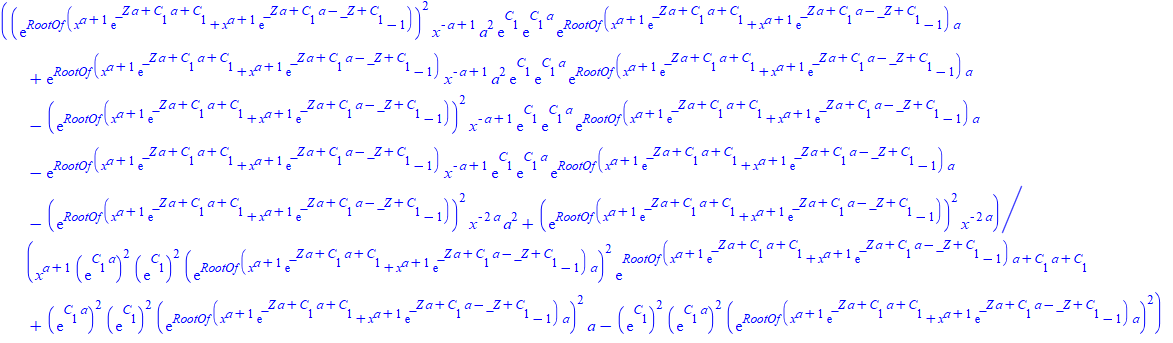Changing C to _C1 gives zero

```restart;
ode:=x*(x-a*y(x))*diff(y(x),x) = y(x)*(y(x)-a*x);
mysol2:=y(x) = exp((-a + 1)*RootOf(x*exp(ln(x)*a + _Z*a + _C1*a + _C1) + exp(ln(x)*a + _Z*a + _C1*a - _Z + _C1)*x - 1) - ln(x)*a - _C1*(a + 1)):
odetest(mysol2,ode);

0```

Example 6

```restart;
ode:=x*diff(y(x),x)^2-a*y(x)*diff(y(x),x)+b = 0:
mysol1:=y(x) = x*RootOf(2*x*_Z^2*a - 2*C*_Z^((2*a - 1)/(a - 1))*a - x*_Z^2 + C*_Z^((2*a - 1)/(a - 1)) - b)/a + b/(RootOf(2*x*_Z^2*a - 2*C*_Z^((2*a - 1)/(a - 1))*a - x*_Z^2 + C*_Z^((2*a - 1)/(a - 1)) - b)*a):
odetest(mysol1,ode);
```

gives many pages of output. Changing C to _C1 gives zero

```restart;
ode:=x*diff(y(x),x)^2-a*y(x)*diff(y(x),x)+b = 0:
mysol2:=y(x) = x*RootOf(2*x*_Z^2*a - 2*_C1*_Z^((2*a - 1)/(a - 1))*a - x*_Z^2 + _C1*_Z^((2*a - 1)/(a - 1)) - b)/a + b/(RootOf(2*x*_Z^2*a - 2*_C1*_Z^((2*a - 1)/(a - 1))*a - x*_Z^2 + _C1*_Z^((2*a - 1)/(a - 1)) - b)*a):
odetest(mysol2,ode);

0```

and more. I think the above should hopefully help locate the issue.

Maple 2020.1 with Physics 708

## Error, (in SolveTools:-CancelInverses) a...

Maple 2020

I see this error once in a while

Error, (in SolveTools:-CancelInverses) assertion failed, simplify should not leak _Z variables out from RootOfs

When I run a long script. Unfortunately, I have not been able to make a small example to reproduce it. So it is random. I've seen it about 5-6 times in the last 2-3 weeks, over 100 runs during this time.

Just wanted to see if anyone saw this before. The strange thing it is random. I can re-run the same script, or restart it from the point where it failed,  with no changes anywhere, and this error do not show up again for days, and suddenly it shows up again.

Must be related how to Maple internal cache memory happened to be arranged at that time. I noticed that a number of things in Maple seem to happen at random times, such as a random hang of the server at different times and places. I could never understand why this happens in Maple.

If there is only one thing Maplesoft could do for the next version, it will be to improve the robustness of its software.

## why Maple gives duplicate solutions from...

Maple 2020

Whenever I call solve, I now make sure to convert the solution to a set first to get rid of duplicate solutions which could be generated.

Is there a way to tell solve to return unique solution only by default?

```restart:
expr:=-1/2*ln(u)-1/4*ln(u^2+2)-ln(x)-C:
sol:=solve(expr = 0,u):
nops([sol])```

8

But there are actually only 4. The other 4 are duplicated

```nops(convert([sol],set))
```

4

This does not happen all the time,but I noticed it few times now. Is this to be expected?

Maple 2020.1

﻿